Confidence Intervals Estimation for Survival Function in Log-Logistic Distribution and Proportional Odds Regression Based on Censored Survival Time Data
Journal of Biometrics & Biostatistics

Like us on:

# Confidence Intervals Estimation for Survival Function in Log-Logistic Distribution and Proportional Odds Regression Based on Censored Survival Time Data

Kamil ALAKUŞ*, Necati Alp ERİLLİ

Ondokuz Mayıs University, Faculty of science and Arts, Department of Statistics, 55139 Turkey

*Corresponding Author:
Kamil ALAKUŞ
Ondokuz Mayıs University
Faculty of science and Arts
Department of Statistics
55139 Turkey
E-mail: [email protected]

Received date: December 21, 2010; Accepted date: June 06, 2011; Published date: September 25, 2011

Citation: ALAKUS K, ERILLI NA (2011) Confidence Intervals Estimation for Survival Function in Log-Logistic Distribution and Proportional Odds Regression Based on Censored Survival Time Data. J Biomet Biostat 2:116. doi: 10.4172/2155-6180.1000116

Copyright: © 2011 ALAKUS K, et al. This is an open-access article distributed under the terms of the Creative Commons Attribution License, which permits unrestricted use, distribution, and reproduction in any medium, provided the original author and source are credited.

Visit for more related articles at Journal of Biometrics & Biostatistics

#### Abstract

Log-logistic and Weibull distributions have both accelerated survival time property. The log-logistic distribution has also proportional odds property. Log-logistic distribution has unimodal hazard curve which changes direction. Link [6,7] presented a confidence interval estimate of survival function using Cox\'s proportional hazard model with covariates. Her idea more recently extended by  to the exponential distribution and  to exponential proportional hazard model, respectively. The same idea has been extended to the Weibull proportional hazard regression model by . In this study, it is formed on confidence interval for log-logistic distribution survival function for any values of the time provided that the survival times have a log-logistic distributed random variable. It is also extended the same results to the proportional odds regression. A Real time data and a simulation data examples are also considered in the study for illustration the discussed confidence interval.

#### Keywords

Confidence interval; Hazard function; Point estimation; Survival analysis; Survival function; Log-logistic distribution; Proportional odds regression

#### Introduction

There are two types of estimation for any identity. One is point estimation and the other is confidence interval estimation. In Survival analysis literature, confidence interval estimate for the survival function is not new. Especially confidence interval estimate for the baseline survival function is extensively studied with many authors. For example, for Kaplan-Meier survival function confidence interval estimate is studied using Greenwood formulae by [4,5] and many others. In Cox's proportional hazard model, [6,7] formed log transformed confidence interval for survival function with covariates. Her idea more recently extended by  to the exponential distribution and  to exponential proportional hazard model, respectively. Weibull proportional hazard function is also investigated by . Interval estimate for survival function is generally useful in the analysis of survival or life time data. In this study, symmetric type and proportional odds transformed confidence interval approach is developed for log-logistic survival function without covariates and proportional odds regression survival function with covariates.

The plan of this study is given as follows. In the next section, the log-logistic distribution and proportional odds regression and their important functions are presented. In section 3, it is formed confidence interval estimate for the survival function of log-logistic distribution and proportional odds regression model too. In section 4; it has given a real data example and as an extension of the real data, simulation study for illustrating the proposed method in this study. The study completed with a discussion section.

Log-logistic distribution and proportional odds regression model

Log logistic distribution: The log-logistic distribution has the proportional odds property and the distribution is the natural one to use in conjunction with the proportional odds model. Cox and Oakes  demonstrated that the log-logistic distribution is the only one that shares the accelerated survival time property and proportional odds property. Situations in which the hazard function changes, direction of hazard function can arise. For example, a patient faces an increasing hazard of death in heart transplantation over the first few days or after the transplant, while the body adapts to the new recovers. The hazard then decreases with time as the patient recovers. In situations such as this, a unimodal hazard function may be appropriate.

A particular form of unimodal hazard is the function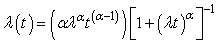(1)

This hazard function decreases monotonically if α = 1. If α> 1, than the hazard has a stable mode. The survival function corresponding to the hazard function in equation (1) is given below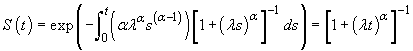(2)

Probability density function is also given below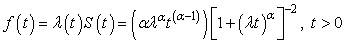(3)

This is the density function of a random variable T which has a log-logistic distribution with parameters α and λ. The distribution is so called because the variable logT has log-logistic distribution and a symmetric distribution whose probability density function is very similar to the normal distribution .

The suitability of the log-logistic distribution for the analysis of a data set can be empirically checked using a linear relationship derived from expression of S(t) and F(t)=1-S(t). The odds of surviving beyond time t are S(t)/F(t)=(λt) and consequently log odds of survival beyond t can be expressed as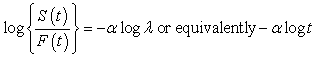or equivalently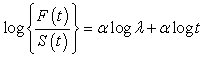(4)

That is, the log-logistic distribution corresponds to a linear model for the log odds of failure over the logarithm of time, with slope α [10,11]. The Kaplan-Meier sample estimate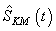can be used to calculate the log odds and a plot of them versus log t should follow approximately a straight line for the log-logistic model in order to be suitable.

Proportional odds regression model

The application of accelerated survival time and proportional odds models to the analysis of reliability data has recently been described by . The general proportional odds model for survival data was introduced by [13,14] describes the proportional odds model.

The log-logistic distribution is commonly extended to include a vector of covariates x by reformulating the survival function is given as follows(5)

Under the accelerated failure time model, the hazard of death at the time of is given below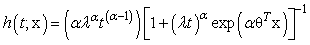(6)

Thus the survival time for the ith observation also has a log-logistic distribution and therefore it has both accelerated failure time and proportional odds property.

Confidence intervals for survival function

Log-logistic distribution: First multiply, both numerator and denominator, by α with equation (2). The equation value will not change. Therefore we can re-write equation (2) as follows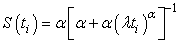(7)

If we take natural logarithm of α(λ)α identity, we can get the result as logα+ α logλ. Now let Ri = logα + logλ be the score (risk score) function. Then we denote logλ with β0 and logα with β1, the score function can be written as Ri = β0 + β1. Therefore the survival function given (7) can be re-written as follows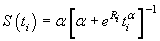(8)

Estimated survival function is also given by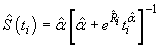(9)

As a result we can prepare for confidence intervals of survival function for the log-logistic distribution. To do this, we first can form confidence intervals for Ri then extended it to the survival function. For the score function Ri 100(1-α)% confidence interval is given by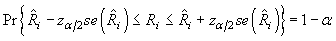(10)

or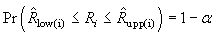(11)

Here Zα/2 denotes coordinate value of standard normal distribution at the significance level of α/2 and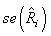is also denotes standard error of estimated score function. The estimated standard error of the score function is calculated using by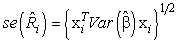(12)

where,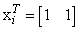is a unit column vector in this simple model and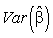is also variance-covariance matrix of estimated parameters.

We can easily form a 100(1-α)% confidence intervals of survival function using score function confidence intervals. So that, the confidence intervals for survival function of a log-logistic distribution are given by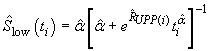for lower limit and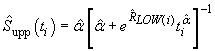for upper limit, respectively. So, 100(1-α)% confidence intervals for survival function of the log-logistic distribution can given as following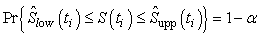(13)

Proportional odds regression model

Let Ri = logα + logλ + αθTxi be score function for i-th observation. If we denote αlogλ with β0 and logα with β1, then the score function for i-th observation can be written as Ri = β0 + β1 + βTxi . Here ßT is (p×1) column vector and equals to αθT.

Let β0 + β1 + βT denote as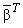a (k×1) size column vector and yi also a same size column vector too. Then the score function for i-th observation can be written as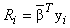. So that, the survival function given in (2) can be written as in equation (14)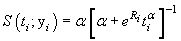(14)

Estimated survival function is also given by equation (15)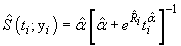(15)

Therefore we can prepare confidence intervals of survival function for the proportional odds regression. As we made for log-logistic distribution, firstly we can form confidence intervals for Ri then extended it to the survival function. For the score function Ri 100(1- α)% confidence interval is given by equation (10) or equation (11). The estimated standard error of the score function for i-th observation is calculated using equation (16)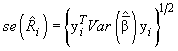(16)

where,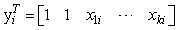a column vector in this model and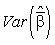is also variance-covariance matrix of estimated parameters. In this model, the estimated variance-covariance matrix might be given as follows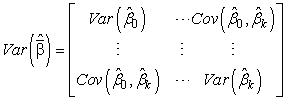(17)

A 100(1-α)% confidence intervals of survival function uses confidence intervals of score function. Namely, the confidence intervals for survival function of a proportional odds model are given by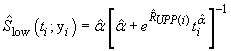for lower limit and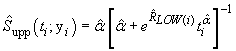for upper limit, respectively. So, 100(1-a)% confidence intervals for survival function of the proportional odds regression can be shown as follows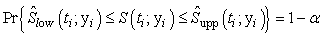(18)

Model selection criterion

In this study we tested performance of proposed model with log-likelihood value and besides with AIC and BIC model selection criterions. The Akaike information criterion (AIC) is a measure of the relative goodness of fit of a statistical model. It was developed by . Bayesian Information Criterion (BIC) is a criterion for model selection among a class of parametric models with different numbers of parameters. It has been introduced by . The AIC and BIC is given as follows, respectively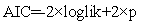(19)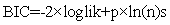(20)

When data has small samples size, corrected AIC can be given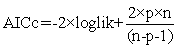(21)

where p is defined as number of free parameters.

Given a set of candidate models for the data, the preferred model is the one with the smallest value of Log Likelihood, AIC and BIC.

Illustrative example

In this section a real data illustration considered to confidence intervals for survival function which we discussed it earlier sections in this study. For this reason, we first give some information about the data just in the next subsection. Second, we use the data for illustrating confidence intervals estimation for the survival function of the proportional odds regression model.

Real time data: Ovarian cancer study

Data are from  on ovarian cancer. The data are taken from . The ovarian cancer frame includes the survival times (in days) and indicator variable (status) of death or censoring plus the following 4 additional variables on each patient. These are patient's age (age), an indicator of the extent of the residual disease (residual.dz), treatment given (rx) and measure of performance score or functional status using the Eastern Cooperative Oncology Group's scale (ecog.ps). The survival analysis chapter in the S-Plus documentation describes these data sets further and illustrates survival analysis methods with them. There were 26 patients in the study. Total censoring ratio is 53.85%.

Confidence intervals for survival function in Proportional odds regression model

We first have taken the goodness of fit for the survival times which comes from a log-logistic distribution. To do this, a common and useful technique for checking the validity of a parametric model is to embed it in larger parametric model and use, e.g., the likelihood ratio test to check whether the reduction to the actual model is valid; for applications in survival analysis [8,19].

Secondly, the tests of the survival times come from a log-logistic distribution. We use the Kolmogorov-Smirnov type test. The test statistic result is D26 = 0.1251. This further indicates that a log-logistic distribution is a reasonable one.

Thirdly, one use of the Nelson-Aalen or Kaplan-Meier estimators for survival data is to check graphically whether the survival time will appear to follow a certain parametric distribution; in fact, this was the ration able behind the estimator in  original paper. For the log-logistic distribution with log odds function isso that log odds plotted against log t should yield an approximately straight line for the log-logistic distribution This result is given in (Figure 1a) for the ovarian cancer data. The curve is roughly linear, suggesting that a model may be appropriate. In the same Figure, the corresponding log odds estimates (straight lines) based on log-logistic distribution are added the graph and it can seen for approximate the Kaplan-Meier estimates quite good too. In (Figure 1b), QQ plot support same results in (Figure 1a). In (Figure 1c) Baseline Hazard function shows unimodal shape. Also in (Figure 1d), proportional odds regression hazard is a increasing function for risk score function of age.

Figure 1: (a) Graphical Test For a Log-Logistic Distribution; (b) QQ Plot; (c) Baseline Hazard Function; (d) odds regression Hazard Function for Ovarian Cancer Study

As a result, (Figure 1d) summarizes that when ages and survival times increases hazard function increases in same direction.

We have also fit the Exponential Hazard Regression (HER), Proportional odds Hazard Regression (POR), Log-Normal Hazard Regression (LNR) and Weibull Hazard Regression (WHR) models to the data in ovarian cancer study. The log likelihood, AIC, AICc and BIC values for each model are given in (Table 1). From the table, we can see that the proportional odds regression model provides the best fits for the data.

Model Log Likelihood Value AIC AICc BIC
EHR -91.7793 185.9986 185.7253 186.8167
POR -89.5509* 183.1018* 183.6235* 185.6180*
LNR -89.7349 183.4698 183.9915 185.9860
WHR -90.0012 184.0024 184.8241 186.5186

Table 1: Results of Fitting Parametric Models to the Ovarian Cancer Data.

As we can see from (Table 1), Proportional odds regression values are smallest for all criterions. Thus we can easily say, Proportional odds regression model is better than the other models.

Therefore we can fit the Proportional odds regression model and the results are given in (Table 2). From the (Table 2), we can see that all three parameters are very significant.

Parameter Value Std. Err. z-Test p-value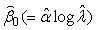-25.9330 3.065 -22.85 0.000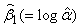0.8003 0.243 3.30 0.000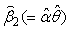0.1975 0.050 3.96 0.001

Table 2: Results of Proportional odds regression Model to the Ovarian Cancer Data.

For calculating the confidence intervals of survival functions estimated variance-covariance matrix is given below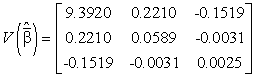Some calculations of confidence intervals for survival function in the log odds regression model is given in (Table 3). Survival probabilities estimates and 95% confidence intervals are also given (Figure 2).

Figure 2: Estimated survival curve with ovarian cancer based on a Proportional odds regression.

Time age95% Confidence Intervals  95% Confidence Intervals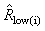Ri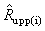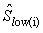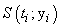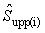59 72.3315 0.752 -12.32502 -10.85052 -9.376026 0.750 0.929 0.983
115 74.4932 0.831 -12.05233 -10.42368 -8.795035 0.275 0.660 0.908
156 66.4658 0.588 -13.16162 -12.00874 -10.85586 0.602 0.827 0.938
421 53.3644 0.691 -15.94933 -14.59569 -13.24205 0.644 0.875 0.964
431 50.3397  0.793 -16.74785 -15.19293 -13.63802 0.718 0.923 0.983
448 56.4301 0.608 -15.18141 -13.99035 -12.79929 0.502 0.769 0.916
464 56.9370 0.597 -15.05972 -13.89026 -12.72080 0.463 0.735 0.900
475 59.8548 0.552 -14.39591 -13.31412 -12.23233 0.335 0.597 0.814
477 64.1753 0.554 -13.54678 -12.46101 -11.37525 0.174 0.385 0.650
563 55.1781 0.638 -15.48886 -14.23756 -12.98627 0.423 0.719 0.899
638 56.7562 0.600 -15.10292 -13.92596 -12.74899 0.304 0.586 0.821
744 50.1096 0.802 -16.80984 -15.23837 -13.66690 0.437 0.789 0.947
769 59.6301 0.554 -14.44461 -13.35849 -12.27237 0.152 0.347 0.611
770 57.0521 0.594 -15.03232 -13.86753 -12.70274 0.215 0.468 0.738
803 39.2712 1.256 -19.84033 -17.37847 -14.91662 0.696 0.964 0.997
855 43.1233 1.085 -18.74517 -16.61785 -14.49054 0.565 0.916 0.989
1040 38.8932 1.273 -19.94846 -17.45311 -14.95777 0.573 0.942 0.995
1106 44.6000 1.022 -18.32942 -16.32627 -14.32312 0.383 0.821 0.971
1129 53.9068 0.674 -15.80995 -14.48859 -13.16723 0.157 0.411 0.724
1206 44.2055  1.039 -18.44022 -16.40417 -14.36811 0.348 0.804 0.969
1227 59.5890 0.555 -14.45356 -13.36660 -12.27965 0.060 0.159 0.359
268 74.5041 0.831 -12.05099 -10.42153 -8.792072 0.054 0.227 0.600
329 43.1370 1.085 -18.74130 -16.61515 -14.48900 0.916 0.989 0.999
353 63.2192 0.546 -13.72033 -12.64980 -11.57927 0.336 0.596 0.812
365 64.4247 0.557 -13.50280 -12.41177 -11.32073 0.266 0.520 0.763
377 58.3096  0.571 -14.73922 -13.61923 -12.49924 0.523 0.771 0.912

Table 3: 95% Confidence Intervals for Survival Probabilities of Proportional odds regression Model to the Ovarian Cancer Data.

Approximate 95% confidence limits are obtained using the risk score function and log odds transformation approach.

Figure 2 shows the log odds regression survival function estimate for ovarian cancer with approximate 95% confidence intervals using (18). Because of small sample data the confidence interval results are little bigger than expected. However we can easily say, the result is quite good.

Simulation study

In order to Ovarian Cancer Data has small sample; to see the behavior of Proportional odds regression on big samples, a simulation study is studied in this sub-section. Simulation study data has 100 variable value and obtained as follows:

Step 1: Mean, variance and standard deviation for age variable in Ovarian Cancer Data is calculated. (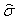=10.10036,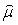= 56.16544)

Step 2: 100 age variable values has been simulated from normal distribution. Here Age = z+where z~N(0,1).

Step 3: 100 random variable value has simulated with uniform distribution in (0,1) interval.

Step 4: With the help of parameter estimation values of Ovarian Cancer Data, survival times is simulated. To do this;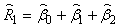Age is evaluated with risk function. Survival time is simulated with the formula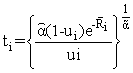. Where,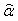=2.22611 and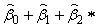are given as in (Table 2).

Step 5: 100 random variable value is simulated with Bernoulli distribution which has mean value (successful probability) p=0.80. Therefore 20% censored survival times is identified using these variables.

The result for simulation data in graphs is given in (Figure 3). In (Figure 3a), the curve is quite linear. In the same Figure, the corresponding log odds estimates (straight lines) based on log-logistic distribution are added the graph and it can seen for approximate the Kaplan-Meier estimates are very good. In (Figure 3b), QQ plot support same results in (Figure 3a). In (Figure 3) Baseline Hazard function shows unimodal shape. Also in (Figure 3d), proportional odds regression hazard is a increasing for risk score function of age.

Figure 3: (a) Graphical Test For a Log-Logistic Distribution; (b) QQ Plot; (c) Baseline Hazard Function; (d) odds regression Hazard Function for Simulation Study.

Table 4 results shows that Proportional odds regression model has the smallest criterion values than the other models. We can easily say that it is the best model above others. In Table 5, estimation of parameters is given and we can say that all estimations are very significant.

Model Log Likelihood Value AIC BIC
EHR -644.8942 1291.788 1294.3936
POR -623.6054* 1251.211* 1256.4211*
LNR -626.3671 1256.734 1261.9445
WHR -643.6997 1291.399 1296.6097

Table 4: Results of Fitting parametric models to the simulated data.-28.9309 1.057 -27.381 0.0000.8687 0.093 9.294 0.0000.2218 0.018 12.492 0.000 Parameter Value Std. Err. z-Test p-value

Table 5: Results of Proportional odds regression Model to the Simulated Data.

For calculating the confidence intervals of survival functions estimated variance-covariance matrix is given below: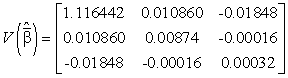In (Figure 4), intervals of simulation data are quite small. Cox-Snell residuals intervals gave robust intervals compared to real time data. Thus we can easily say that when data sample gets bigger, expected intervals will be smaller on survival structural data sets.

Figure 4: Estimated survival curve with simulated data based on a proportional odds regression.

#### Discussion

Many statistical investigations can occur both estimation and hypothesis testing. Estimation can be made in two different types. One is point estimation and the other is interval estimation. Both point and interval estimations can be achieve with an estimator. Interval estimation is generally called confidence interval estimation and naturally the estimators are also called confidence interval estimators.

Survival function may be the most important function in survival analysis or reliability analysis. Probability of living longer than t time is an important issue for both doctors and patients or patient relatives. Researching for factors which affects this issue is also important for determining risk factor function variables on survival times. It is necessary to search influence of hazard function for survival analysis. Both point estimation and confidence interval estimation of the survival function may be achieved by fitting parametric distributions. Semiparametric proportional hazard model is known Cox regression model. In the Cox regression model confidence interval estimation of survival function has studied by Link [6,7]. Her idea more recently extended by Alakus et al.  to the exponential distribution and Alakus et al.  to exponential proportional hazard model, respectively. Weibull proportional hazard function is also investigated by Alakus .

For this reason, in this study we offer a new confidence interval with transformed log odds in symmetric type in proportional odds regression model. Proposed approach is studied with real time data and simulation data. Results were quite good enough. When sample size getting larger, confidence intervals were getting tighter. Model selection criterions Log-Likelihood, AIC and BIC gave the best results for proposed model. Confidence intervals are narrower than other studied models. At the thought real time data has small sample, a larger data set with simulation data also studied. Results are more robust than small sample. Based on the theorem of large numbers law; S(t;y) will be converges in probability to S(t;y). This summarizes us when sample size is quite enough, distribution of risk function approximate to normal distribution and this becomes S(t;y) closer to S(t;y). This is what we are investigating for.

The investigated confidence intervals may be extended to lognormal hazard model. This problem will be investigated by the forthcoming studies.

#### Acknowledgements

The authors are highly thankful to the referees and the editor for their valuable and useful suggestions.

#### References

Select your language of interest to view the total content in your interested language

### Article Usage

• Total views: 13724
• [From(publication date):
September-2011 - Dec 11, 2019]
• Breakdown by view type
• HTML page views : 9842Can't read the image? click here to refresh